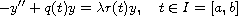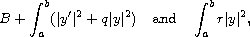Electron. J. Differential Equations, Vol. 2016 (2016), No. 314, pp. 1-7.

### Complex oscillations of non-definite Sturm-Liouville problems Mervis Kikonko, Angelo B. Mingarelli

Abstract:
We expand upon the basic oscillation theory for general boundary problems of the formwhere q and r are real-valued piecewise continuous functions and y is required to satisfy a pair of homogeneous separated boundary conditions at the end-points. The non-definite case is characterized by the indefiniteness of each of the quadratic formsover a suitable space where B is a boundary term. In 1918 Richardson proved that, in the case of the Dirichlet problem, if r(t) changes its sign exactly once and the boundary problem is non-definite then the zeros of the real and imaginary parts of any non-real eigenfunction interlace. We show that, unfortunately, this result is false in the case of two turning points, thus removing any hope for a general separation theorem for the zeros of the non-real eigenfunctions. Furthermore, we show that when a non-real eigenfunction vanishes inside I, the absolute value of the difference between the total number of zeros of its real and imaginary parts is exactly 2.

Submitted September 12, 2016. Published December 10, 2016.
Math Subject Classifications: 34C10, 34B25.
Key Words: Sturm-Liouville; non-definite; indefinite; spectrum; oscillation; Dirichlet problem; turning point.

Show me the PDF file (188 KB), TEX file for this article.Mervis Kikonko Department of Engineering Sciences and Mathematics Lulea University of Technology SE-971 87 Lule{\aa}, Sweden email: mervis.kikonko@ltu.se, mervis.kikonko@unza.zm Angelo B. Mingarelli School of Mathematics and Statistics Carleton University Ottawa, ON, Canada, K1S 5B6 email: angelo@math.carleton.ca